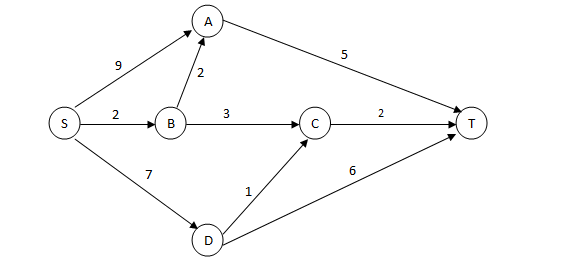# Logical Reasoning Puzzles for CAT

0
24116

Puzzles is one of the important topic in the logical reasoning section of CAT exam. Puzzles test your logical, analytical and mathematical ability in approaching a problem. The approach and technique will be hidden in the problem itself. To improve your puzzle solving you need to solve more puzzles and more-ever you need to try the puzzle on your own before following the given solution. Even a small simple mathematical/reasoning concept can be used to frame CAT puzzles. The CAT puzzle we have given here is based on the Routes and Networks. Download all Logical reasoning questions for CAT PDF.

Logical Reasoning Puzzles for CAT:

Instructions:

A significant amount of traffic flows from point S to point T in the one-way street network shown below. Points A, B, C, and D are junctions in the network, and the arrows mark the direction of traffic flow. The fuel cost in rupees for travelling along a street is indicated by the number adjacent to the arrow representing the street. –Motorists traveling from point S to point T would obviously take the route for which the total cost of traveling is the minimum. If two or more routes have the same least travel cost, then motorists are indifferent between them. Hence, the traffic gets evenly distributed among all the least cost routes.

The government can control the flow of traffic only by levying appropriate toll at each junction. For example, if a motorist takes the route S-A-T (using junction A alone), then the total cost of travel would be Rs 14 (i.e., Rs 9 + Rs 5) plus the toll charged at junction A.

Question 1:

If the government wants to ensure that all motorists travelling from S to T pay the same amount (fuel costs and toll combined) regardless of the route they choose and the street from B to C is under repairs (and hence unusable), then a feasible set of toll charged (in rupees) at junctions A, B, C, and D respectively to achieve this goal is:

A. 2,5,3,2

B. 0,5,3,2

C. 1,5,3,2

D. 2,3,5,1

Question 2:

If the government wants to ensure that no traffic flows on the street from D to T, while equal amount of traffic flows through junctions A and C, then a feasible set of toll charged (in rupees) at junctions A, B, C,and D respectively to achieve this goal is:

A. 1,5,3,3

B. 1,4,4,3

C. 0,5,2,2

D. 0,5,2,3

Question 3:

If the government wants to ensure that all routes from S to T get the same amount of traffic, then a feasible set of toll charged (in rupees) at junctions A, B, C, and D respectively to achieve this goal is:

A. 0, 5, 2, 2

B. 0,5,4,1

C. 1,5,3,3

D. 1, 5, 3,2

Question 4:

If the government wants to ensure that the traffic at S gets evenly distributed along streets from S to A, from S to B, and from S to D, then a feasible set of toll charged (in rupees) at junctions A, B, C, and D respectively to achieve this goal is:

A. 0,5,4,1

B. 0,5,2,2

C. 1,5,3,3

D. 1,5,3,2

Question 5:

The government wants to devise a toll policy such that the total cost to the commuters per trip is minimized. The policy should also ensure that not more than 70 per cent of the total traffic passes through junction B. The cost incurred by the commuter travelling from point S to point T under this policy will be:

A. Rs. 7

B. Rs. 9

C. Rs. 10

D. Rs. 13

Solutions for Logical reasoning CAT puzzle – Routes and Networks:

Let the toll charged at junctions A, B, C, and D be a,b,c and d respectively. Then the so that equal amount is collected through all route we have, 9+a+5=2+b+2+a+5=10+d+c=13+d. Then from the options only option C satisfies the above equality. hence option C.

Let the toll charged at junctions A, B, C, and D be a,b,c and d respectively. Now since we want equal traffic through A and C , total cost through routes passing from A and C should be equal. So we have (9+a+5) + (2+b+2+a+5) = (2+3+b+c+2) + (7+d+1+c+2) . Only option E satisfy the above equality.

Now  the fuel cost along different routes are :
SAT = 14
SBAT = 9
SBCT = 7
SDCT = 10
SDT = 13
Now , if we consider option D . Total cost for all routes comes out to be same which is 15. Hence option D.

Total cost = fuel cost + toll
Total cost along SAT : 14+tollA
Total cost along SBAT : 9+tollA+tollB
Total cost along SDT : 13+tollD
Now when option A is considered , total costs come out to be same.
​Hence option A is correct